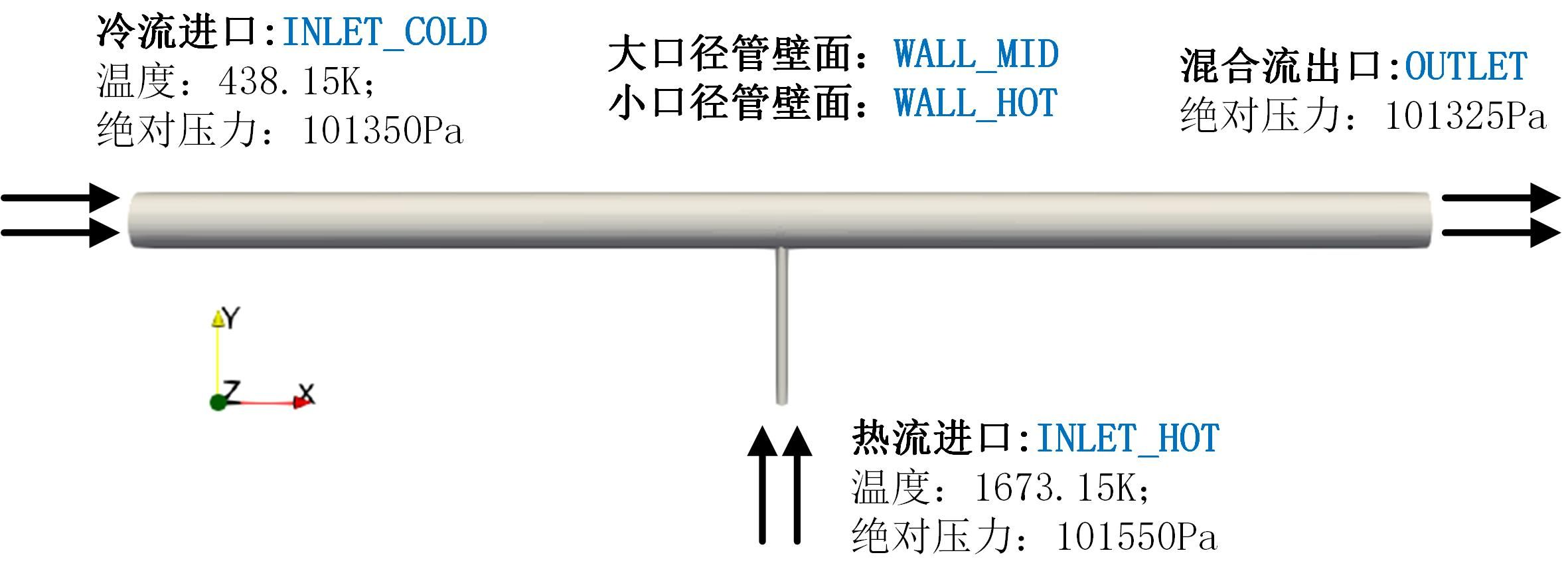## rhoPimpleFoam解析

##### 1. 引言##### 2. 控制方程与算法

\begin{equation} \frac{\partial \rho}{\partial t}+\nabla \cdot \left(\rho \mathbf{U} \right)=0 \label{NS1} \end{equation} \begin{equation} \frac{\partial \rho \mathbf{U} }{\partial t}+\nabla \cdot \left(\rho \mathbf{U} \mathbf{U} \right)-\nabla \cdot \tau=-\nabla p \label{NS2} \end{equation} \begin{equation} \frac{\partial \rho h}{\partial t}+\nabla \cdot (\rho \mathbf{U} h) + \frac{\partial \rho K}{\partial t}+\nabla \cdot (\rho \mathbf{U} K) - \nabla \cdot (\alpha_\mathrm{eff}\nabla h) =\frac{\partial p}{\partial t} \label{hfinal} \end{equation}

###### 2.1. 密度方程与速度方程

\begin{equation} \int \int {\frac{{\partial\rho \mathbf{U}}}{{\partial t}}\mathrm{d}V\mathrm{d}t = \left( {\rho_\rP^{t+\Delta t}\mathbf{U}_\mathrm{P}^* -\rho_\rP^{t} \mathbf{U}_\mathrm{P}^t} \right)\;} {V_\mathrm{P}}, \label{mom1} \end{equation}

\begin{equation} \int \int {\nabla \cdot \left( {\rho\mathbf{U}\mathbf{U}} \right)\mathrm{d}V\mathrm{d}t = \int \int {\rho\mathbf{U}\mathbf{U}\cdot\mathrm{d}\bfS\mathrm{d}t} = } \sum {{{\left( {{\rho^t\mathbf{U}^*}{\mathbf{U}^t}} \right)}_f}} \cdot\bfS_f\Delta t = \Delta t\sum {{F_f^t \mathbf{U}_f^*} } , \label{mom2} \end{equation}

\begin{equation} -\int \int \nabla p \mathrm{d}V\mathrm{d}t=-\int\int p \mathrm{d}\bfS\mathrm{d}t=-\Delta t\sum \left(p_f^t\bfS_f\right), \label{gradp} \end{equation}

\begin{equation} \left( {\rho_\rP^{t+\dt}\mathbf{U}_\mathrm{P}^* -\rho_\rP^t \mathbf{U}_\mathrm{P}^t} \right)\; {V_\mathrm{P}} + \Delta t\sum F_f^t \mathbf{U}_f^* =-\Delta t\sum \left(p_f^t\bfS_f\right) \label{predic} \end{equation}

\begin{equation} \mathbf{U}_f^* = \frac{\bfU_\rP^* + \bfU_\rN^*}{2}. \label{linear} \end{equation}
\begin{equation} p_f^t = \frac{p_\mathrm{P}^t + p_\mathrm{N}^t}{2}. \label{linear2} \end{equation}

\begin{equation} \left( {\rho_\rP^{t+\dt}\mathbf{U}_\mathrm{P}^* -\rho_\rP^t \mathbf{U}_\mathrm{P}^t} \right)\; {V_\mathrm{P}} + \Delta t\sum F_f^t \frac{\bfU_\rP^* + \bfU_\rN^*}{2} =-\Delta t\sum \left(\frac{p_\mathrm{P}^t + p_\mathrm{N}^t}{2}\bfS_f\right) \end{equation}

\begin{equation} \left(\frac{\rho_\rP^{t+\dt}}{\Delta t}+\frac{\sum F_f^t}{2V_\rP} \right)\bfU_\rP^* + \frac{\sum F_f^t}{2V_\rP}\bfU_\rN^* = \frac{\rho_\rP^{t}}{\Delta t}\bfU_\rP^t-\frac{1}{V_\rP}\sum \left(\frac{p_\mathrm{P}^t + p_\mathrm{N}^t}{2}\bfS_f\right) \label{predic2} \end{equation}

\begin{equation} {A_\mathrm{P}}\mathbf{U}_\mathrm{P}^*{\rm{ + }}\sum {A_\mathrm{N}\mathbf{U}_\mathrm{N}^*} = S_\mathrm{P}^t-\frac{1}{V_\rP}\sum \frac{{p_\mathrm{P}^t + p_\mathrm{N}^t}}{2}\bfS_f, \label{apanmom} \end{equation}

###### 2.2. 压力泊松方程

\begin{equation} \bfHbyA_\rP^{*} = \frac{1}{{{A_\mathrm{P}}}}\left( { - \sum {{A_\mathrm{N}}\mathbf{U}_\mathrm{N}^{*}} + S_\rP^t} \right) \label{hbya2} \end{equation}

\begin{equation} \bfU_\rP^{*}=\bfHbyA_\rP^{*} - \frac{1}{{{A_\mathrm{P}}}} \frac{1}{V_\rP}\sum_f p_f^{t}\bfS_f \label{Up2} \end{equation}
\begin{equation} \bfU_f^{*}=\bfHbyA_f^{*} - \frac{1}{{{A_{\mathrm{P},f}}}} \left(\frac{1}{V_\rP}\sum_f p_f^{t}\bfS_f\right)_f \label{Up3} \end{equation}

\begin{equation} \frac{\rho^{t+\dt}-\rho^t}{\dt}+\sum_f (\rho_f^t+\rho_f')(\mathbf{U}_f^{*}+\bfU_f') \cdot \bfS_f=0. \label{cont0} \end{equation}

\begin{equation} \frac{\rho^{t+\dt}-\rho^t}{\dt}+\sum_f \left(\rho_f^t\bfU_f^{*} + \rho_f^n\bfU_f' + \frac{1}{RT}p_f'\bfU_f^{*} \right)\cdot\bfS_f=0. \label{cont} \end{equation}

\begin{equation} \bfU_\rP^{t+\dt}=\bfHbyA_\rP^{*} - \frac{1}{{{A_\mathrm{P}}}} \frac{1}{V_\rP}\sum_f p_f^{t+\dt}\bfS_f \label{Up22} \end{equation}

\begin{multline} \frac{\rho^{t+\dt}-\rho^t}{\dt} \\ +\sum_f \left(\rho_f^t\left(\bfHbyA_f^{*} - \frac{1}{{{A_{\mathrm{P},f}}}} \left(\frac{1}{V_\rP}\sum_f p_f^{t}\bfS_f\right)_f\right) - \rho_f^n\frac{1}{V_\rP}\frac{1}{{{A_f^{n}}}} \left(\sum_f p_f’\bfS_f\right)_f + \frac{1}{RT}p_f'\bfU_f^{*} \right)\cdot\bfS_f \\ =0. \label{cont2} \end{multline}

\begin{equation} \frac{\rho^{t+\dt}-\rho^t}{\dt}+\sum_f \rho_f^n \mathbf{HbyA}^{*}_f\cdot\bfS_f + \sum_f \frac{1}{RT}p_f'\bfU_f^{*}\cdot\bfS_f = \sum_f \left(\frac{\rho_f^n}{{{A_f^n}}} \left(\frac{1}{V_\rP}\sum_f p_f^{t+\dt}\bfS_f\right)_f \right)\cdot\bfS_f \label{press} \end{equation}

\begin{equation} \frac{\rho^{t+\dt}-\rho^t}{\dt}+\sum_f \rho_f^n \mathbf{HbyA}^{*}_f\cdot\bfS_f = \sum_f \left(\frac{\rho_f^n}{{{A_f^n}}} \left(\frac{1}{V_\rP}\sum_f p_f^{t+\dt}\bfS_f\right)_f \right)\cdot\bfS_f \label{pres} \end{equation}

\begin{equation} \frac{\p\rho}{\p t}+\nabla \cdot \left(\rho\mathbf{HbyA} \right)=\nabla \cdot \left( \frac{\rho}{{{A}}}\nabla p \right) \label{p2pre} \end{equation}

1. 依据初始速度组建通量，显性离散方程\eqref{NS1}，获得下一个时间步的密度$\rho^{t+\dt}$；
2. 通过方程\eqref{apanmom}，获得$\bfU^*$以及$\mathbf{HbyA}^*$；
3. 求解方程\eqref{pres}，获得压力$p^{t+\dt}$，注意，$p^{t+\dt}$并不是精准的；
4. 通过方程\eqref{Up22}获得速度$\bfU^{t+\dt}$以及通量，在此通过方程\eqref{NS1}更新密度$\rho^{t+\dt}$。再次注意，注意，$\bfU^{t+\dt}$并不是精准的；
5. 依据状态方程，以及压力$p^{t+\dt}$，更新$\rho^{t+\dt}$，通过$\rho^*$与$\rho^{t+\dt}$，求得连续性误差；
6. 回到第一步迭代求解几次，在时间步内收敛的情况下，$\rho^* \rightarrow \rho^{t+\dt}$；

##### 3. 验证算例
###### 3.1 翼型

rhoPimpleFoam自带若干算例。在OpenFOAM-6中，自带的算例主要为以下几个：

• aerofoilNACA0012：马赫数约为0.6的可压缩流动；
• cavity：附加传热的顶盖驱动流；
• helmholtzResonance：可压缩流动模拟亥姆霍兹共振；

###### 3.1. 模型设置

• 初始场p：内部即均一分布uniform 1e5；注意可压缩流体求解器压力为真实的压力值，不可压缩流体的压力为相对压力值。边界场采用自由流边界；
• 初始场速度U：内部场速度以及边界场速度均为均一分布：uniform (600 0 30)；边界场采用超音速自由流边界条件；
• 边界场T：内部场为uniform 298；壁面为零法向梯度；进口采用固定值边界条件，出口采用零法向梯度；

constant文件夹的物性主要设置如下：

• 粘度模型选择层流，即laminar
• 热动力库进行空气的标准指定：
mixture
{
specie
{
molWeight   28.9;//摩尔分数
}
thermodynamics
{
Cp          1005;//比热
Hf          0;//单组分为0
}
transport
{
mu          0;//粘度
Pr          0.71;//普朗特数
}
}
其中mu指定为0，即无粘。详细设置不便于在此处一一说明，可下载算例文件查看。
###### 3.2. 算例运行

./Allrun###### 3.2 掺合阀• 对结果显示截面，可以看出小圆管下方的湍流粘度被高估导致流场存在一系列错误的特征。这可以通过调节网格、湍流模型、以及边界条件来改善；
• 本算例网格由于小圆管的存在导致网格需要特殊处理，如多个O网格、渐变处理等。实际上本算例并不需要单独画出小圆管。单独将大圆管画出，并在壁面上扣除一个洞当做进口边界条件可以大幅度的降低网格数量同时提高网格质量。在这种情况下，上述高度湍流粘度的问题将大幅度改善；
• 初步结果表明本算例可以达到一个稳态的流动状态，因此使用稳态求解器如rhoSimpleFoam会更具有计算经济性；